# 例子

• 需求
有一个学生列表，要求提取分数在90.0或以上的学生，并按分数升序对他们进行排序
• 不使用stream流
``````        List<Student> students=new ArrayList<>();
List<Student> studentsScore = new ArrayList<Student>();
for(Student s : students) {
if(s.getScore() >= 90.0) {
}
}
Collections.sort(studentsScore, new Comparator<Student>() {
@Override
public int compare(Student s1, Student s2) {
return Double.compare(
s1.getScore(), s2.getScore());
}
});
``````
• Stream流
``````List<Student> studentsScore = students
.stream()
.filter(s -> s.getScore() >= 90.0)
.sorted(Comparator.comparing(Student::getScore))
.collect(Collectors.toList());
``````

Stream流的书写非常简洁、清晰

# 什么是Stram流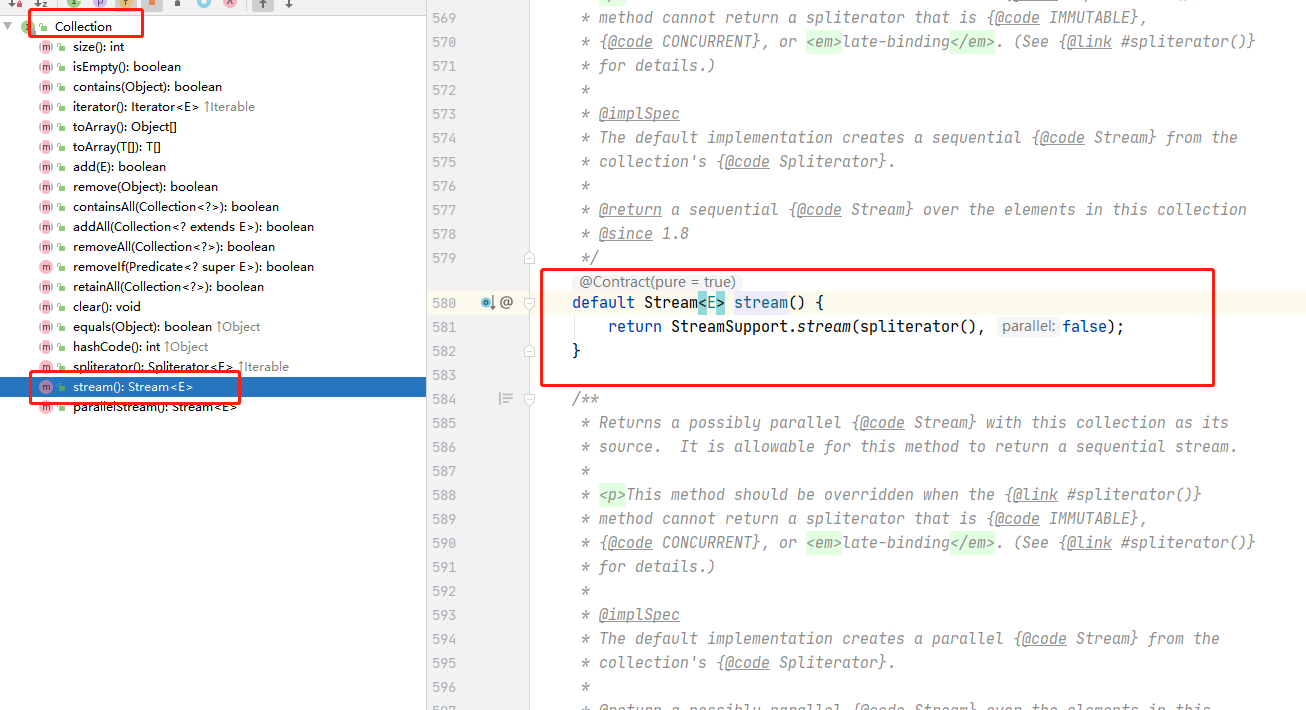Collecion接口的stram的默认default方法返回一个Stram流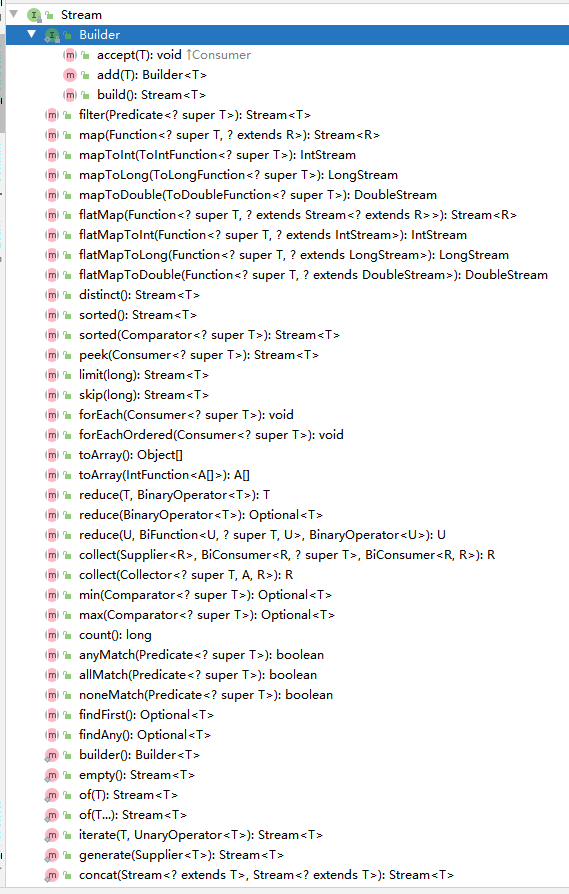## Stram流的特征

• 流不存储它的元素
元素存储在一个集合中，或者动态生成。它们只能通过一系列操作管道从源头进行传输
• 流是不可变的
流不会改变它们的基本元素来源。例如，如果从流中删除某个元素，则创建一个新的流，删除该元素
• 流是不可重用的
流只能穿越一次。在执行终端操作之后(稍后我们将看到这意味着什么) ，您必须从源创建另一个流来进一步处理它
• 流不支持对其元素进行索引访问
流不是集合或数组。你能做的最多就是得到他们的第一个元素。
• 可以使流并发地执行其操作，而不必编写任何多线程代码
• 流是惰性的
尽可能推迟其操作的执行，直到需要结果或者数据
• 允许这种懒惰的一个因素是他们的操作设计方式。它们中的大多数返回一个新的流，允许将操作链接起来并形成一个支持这种优化的管道。

## Stream流的使用过程

• 创建Stream
• 应用0或多个中间操作将初始Stream转换为新的Stream
• 应用终止方法得到结果

# 创建Stream

Stream流由java.util.stream.Stream < t > 接口表示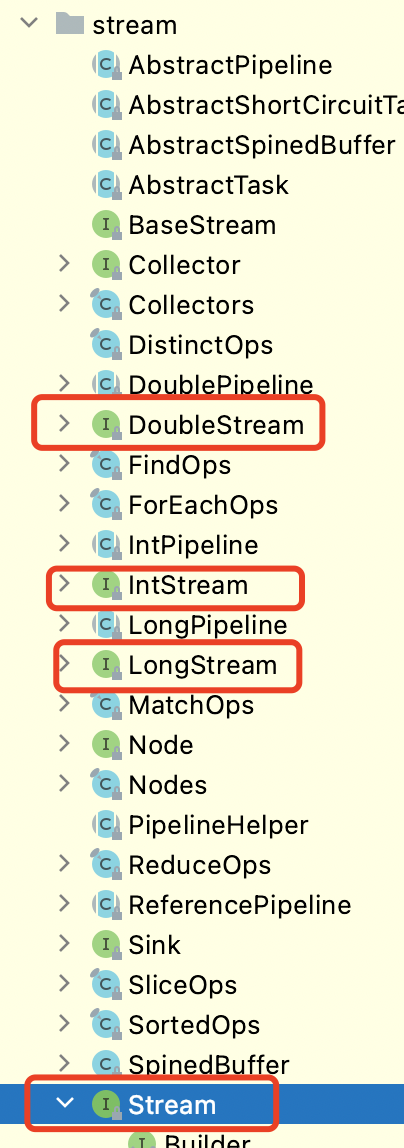## Collection接口

• 从Collection接口的实现类使用默认default方法stream()创建
``````default Stream<E> stream() {
return StreamSupport.stream(spliterator(), false);
}
``````
``````List<String> words = Arrays.asList(new String[]{"hello", "hola", "hallo", "ciao"});
Stream<String> stream = words.stream();
``````

## Stream接口的可变参数

• 从Stream接口的静态static方法of(args)方法创建
``````public static<T> Stream<T> of(T... values) {
return Arrays.stream(values);
}
``````
``````Stream<String> stream = Stream.of("hello","hola", "hallo", "ciao");
``````

## Stream接口的可变参数（从一个数组创建一个流）

• 也是Stream接口的同一个静态方法，入参不同
``````String[] words = {"hello", "hola", "hallo", "ciao"};
Stream<String> stream = Stream.of(words);
``````

## 特别注意

``````int[] nums = {1, 2, 3, 4, 5};
Stream.of(nums)
System.out.println(Stream.of(nums).count()); // It prints 1!
``````
``````// returns a stream of one element
static <T> Stream<T> of(T t)
// returns a stream whose elements are the specified values
static <T> Stream<T> of(T... values)
``````
• 原因
上述返回的是一个流里面包含一个数组元素，而不是5个int元素。因为int不是对象，而int[]是对象，所以创建stream流的方法是第一个静态方法
• 解决
1.我们可以强制 Java 通过创建一个对象数组(带 Integer)来选择 vargs 版本:
``````Integer[] nums = {1, 2, 3, 4, 5};
// It prints 5!
System.out.println(Stream.of(nums).count());
``````

2.或者使用其他方式创建stream流

``````int[] nums = {1, 2, 3, 4, 5};
// It also prints 5!
System.out.println(Arrays.stream(nums).count());
``````
`````` public static IntStream stream(int[] array) {
return stream(array, 0, array.length);
}
``````

3.或者使用InsStream，其实也是调用Arrays.stream(nums)方法

``````int[] nums = {1, 2, 3, 4, 5};
// It also prints 5!
System.out.println(IntStream.of(nums).count());
``````
``````public static IntStream of(int... values) {
return Arrays.stream(values);
}
``````

## 创建流的其他方法

### 生成无限流generate

``````static <T> Stream<T> generate(Supplier<T> s)
``````

``````Stream<T> limit(long maxSize)
``````

``````System.out.println(IntStream.of(nums2).count());
Stream<Double> s = Stream.generate(new Supplier<Double>() {
@Override
public Double get
() {
return Math.random();
}
}).limit(5);
System.out.println(s.collect(Collectors.toList()));
````````````Stream<Double> s = Stream.generate(() -> Math.random()).limit(5);
``````

``````Stream<Double> s = Stream.generate(Math::random).limit(5);
``````

### 生成无限流iterate

``````static <T> Stream<T> iterate(T seed, UnaryOperator<T> f)
``````

``````Stream<Integer> s = Stream.iterate(1, new UnaryOperator<Integer>() {
@Override
public Integer apply(Integer t) {
return t * 2; }
}).limit(5);
``````

``````Stream<Integer> s = Stream.iterate(1, t -> t * 2).limit(5);
``````

### 内部public接口Stream.Builder

Stream.Builder类遵循构造器模式，拥有向正在构建的流添加元素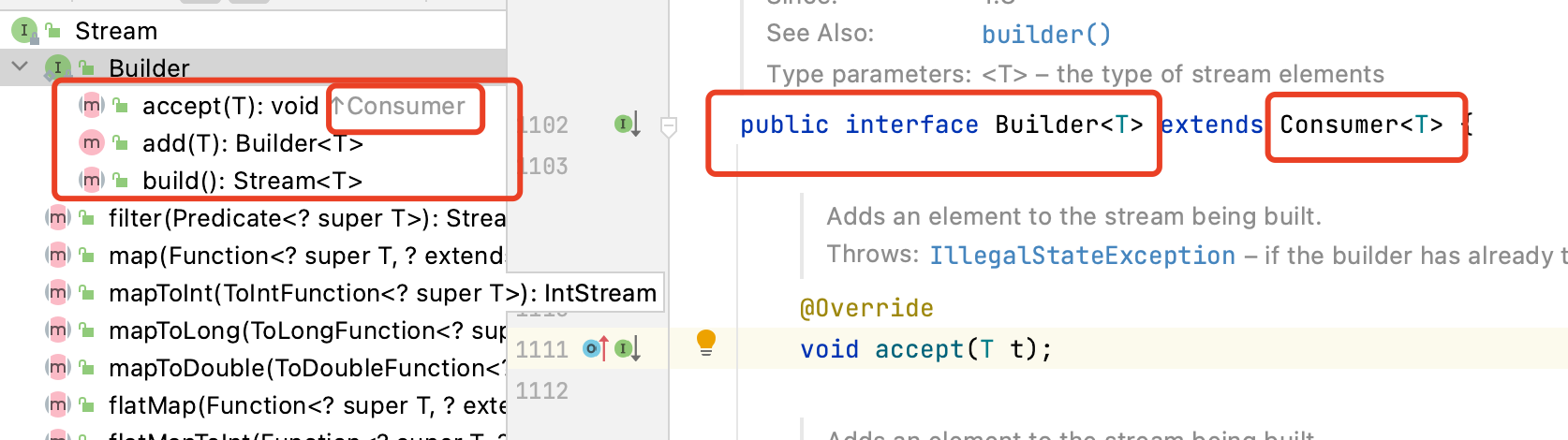``````void accept(T t)
IntStream build();
``````
``````Stream.Builder<String> builder = Stream.<String>builder().add("h").add("e").add("l").add("l");
builder.accept("o");
Stream<String> s = builder.build();
``````

### IntStream 和 LongStream

• 其定义了静态static方法返回 int 或 long 元素范围的顺序流
``````static IntStream range(int startInclusive, int endExclusive)
static IntStream rangeClosed(int startInclusive, int endInclusive)
static LongStream range(long startInclusive, long endExclusive)
static LongStream rangeClosed(long startInclusive, long endInclusive)
``````
``````// stream of 1, 2, 3
IntStream s = IntStream.range(1, 4);
// stream of 1, 2, 3, 4
IntStream s = IntStream.rangeClosed(1, 4);
``````

# 中间操作

• 中间操作总是返回一个新的流
``````Stream<String> s = Stream.of("m", "k", "c", "t")
.sorted()
.limit(3)
``````

• 无状态操作在处理新元素时不保留以前元素的状态，因此可以独立于对其他元素的操作来处理每个元素。
• 有状态操作（如distinct和sorted），在处理新元素时，可能会合并以前看到的元素的状态。

## 有状态操作

• Stream distinct()
返回由不同元素组成的流
• Stream limit(long maxSize)
返回被截断为不长于的maxSize长度的流
• Stream skip(long n)
在丢弃前 n 个元素之后，返回一个包含此流剩余元素的流
• Stream sorted()
返回根据元素的自然顺序排序的流
• Stream sorted(Comparator<? super T> comparator)
返回根据Comparator排序的流

## 无状态操作

• Stream filter(Predicate<? super T> predicate)
返回与给定谓词匹配的元素流
• Stream flatMap(Function<? super T,? extends Stream<? extends R>> mapper)
返回一个包含通过将提供的映射函数应用于每个元素而生成的内容的流
• Stream map(Function<? super T,? extends R> mapper)
返回一个流，其中包含将给定函数应用于此流的元素的结果
• Stream peek(Consumer<? super T> action)
返回包含此流元素的流，对每个元素执行提供的操作

# 终止操作

``````int[] digits = {0, 1, 2, 3, 4 , 5, 6, 7, 8, 9};
IntStream s = IntStream.of(digits);
long n = s.count();
System.out.println(s.findFirst()); // An exception is thrown
``````

## 终止操作方法

• boolean allMatch(Predicate<? super T> predicate)
• boolean anyMatch(Predicate<? super T> predicate)
• boolean noneMatch(Predicate<? super T> predicate)
• Optional findAny()
• Optional findFirst()
• <R,A> R collect(Collector<? super T,A,R> collector)
• long count()
• void forEach(Consumer<? super T> action)
• void forEachOrdered(Consumer<? super T> action)
• Optional max(Comparator<? super T> comparator)
• Optional min(Comparator<? super T> comparator)
• T reduce(T identity, BinaryOperator accumulator)
• Object[] toArray()
• < A > A[] toArray(IntFunction<A[]> generator)
• Iterator iterator()
• Spliterator spliterator()

# 惰性操作

``````//生成一个Stream<String>
Stream.of("sun", "pool", "beach", "kid", "island", "sea", "sand")
//转换为Stream<Integer>
.map(str -> str.length())
//过滤Integer大于3
.filter(i -> i > 3)
//获取流的前2个元素
.limit(2)
//打印流
.forEach(System.out::println);
``````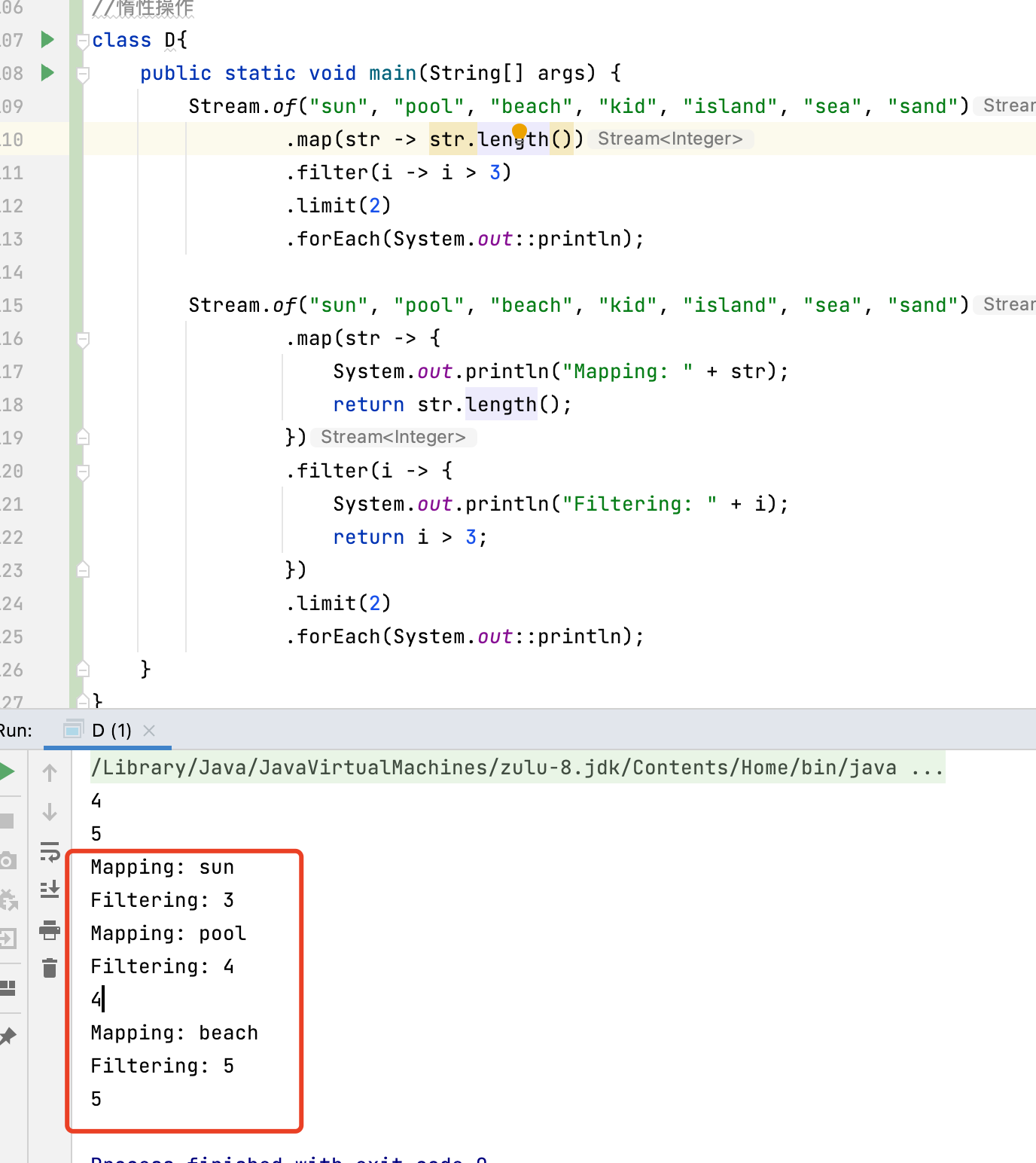# 总结

• 流与lambda完美结合。
• 流不存储其元素。
• 流是不变的。
• 流不可重用。
• 流不支持对其元素的索引访问。
• 流很容易并行化。
• 如果可能的话，流操作是懒惰的。
• 流操作可以链接起来形成一个管道。要设置此管道，请执行以下操作：
1.创建流。
2.应用零个或多个中间操作将初始流转换为新流。
3.应用终端操作生成结果或“副作用”。
• 常用的创建Stream的方法有
``````// From an existing collection
List<String> words = Arrays.asList(new String[]{"hello", "hola", "hallo", "ciao"});
Stream<String> s1 = words.stream();
// From individual elements
Stream<String> s2 = Stream.of("hello", "hola", "hallo", "ciao");
// From an array
String[] words = {"hello", "hola", "hallo", "ciao"};
Stream<String> s3 = Stream.of(words);
``````
• 使用基本类型时不要使用Stream.of（）。而是使用Arrays.stream或stream的基本版本：
``````int[] nums = {1, 2, 3, 4, 5};
IntStream s1 = Arrays.stream(nums);
IntStream s2 = IntStream.of(nums);
``````
• 中间操作（如map或filter）总是返回一个新的流，并被分为无状态操作和有状态操作，它们是惰性的，这意味着它们被推迟到终端操作被调用。
• 诸如count或forEach之类的终端操作总是返回流以外的内容。
• 短路操作导致处理中间操作，直到产生结果。
• 这样，由于延迟和短路操作，流不会对其所有元素执行所有操作，而是直到可以推断或生成结果为止。